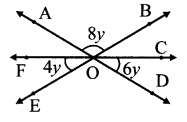# Maharashtra Board Practice Set 21 Class 7 Maths Solutions Chapter 4 Angles and Pairs of Angles

Balbharti Maharashtra State Board Class 7 Maths Solutions covers the 7th Std Maths Practice Set 21 Answers Solutions Chapter 4 Angles and Pairs of Angles.

## Angles and Pairs of Angles Class 7 Practice Set 21 Answers Solutions Chapter 4

Question 1.
∠ACD is an exterior angle of ∆ABC. The measures of ∠A and ∠B are equal. If m∠ACD = 140°, find the measures of the angles ∠A and ∠B.Solution:
Let the measures of ∠A be x°.
m∠A = m∠B = x°
∠ACD is the exterior angle of ∆ABC
∴ m∠ACD = m∠A + m∠B
∴ 140 = x + x
∴ 140 = 2x
∴ 2x = 140
∴ x = $$\frac { 140 }{ 2 }$$
= 70
∴ The measures of the angles ∠A and ∠B is 70° each.

Question 2.
Using the measures of the angles given in the figure alongside, find the measures of the remaining three angles.Solution:
m∠EOD = m∠AOB = 8y ….(vertically opposite angles)
∠FOL, ∠EOD and ∠COD form a straight angle.
∴ m∠FOE + m∠EOD + m∠COD = 180°
∴ 4y + 8y + 6y = 180
∴ 18y = 180
∴ y = $$\frac { 180 }{ 18 }$$
∴ y = 10
m∠EOD = 8y = 8 x 10 = 80°
m∠AOF = m∠COD ….(Vertically opposite angles)
= 6y = 6 x 10 = 60°
m∠BOC = m∠FOE ….(Vertically opposite angles)
= 4y = 4 x 10 = 40°
∴ The measures of ∠EOD, ∠AOF and ∠BOC are 80°, 60° and 40° respectively.

Question 3.
In the isosceles triangle ABC, ∠A and ∠B are equal. ∠ACD is an exterior angle of ∆ABC. The measures of ∠ACB and ∠ACD are (3x – 17)° and (8x + 10)° respectively. Find the measures of ∠ACB and ∠ACD. Also find the measures of ∠A and ∠B.Solution:
Let the measure of ∠A be y°. A
∴ m∠A = m∠B = y°
∠ACB and ∠ACD form a pair of linear angles.
∴ m∠ACB + m∠ACD = 180°
∴ (3x – 17) + (8x + 10) = 180
∴ 3x + 8x – 17 + 10 = 180
∴ 11x – 7 = 180
∴ 11x – 7 + 7 = 180 + 7 …(Adding 7 on both sides.)
∴ 11x = 187
∴ x = $$\frac { 187 }{ 11 }$$ = 17
m∠ACB = 3x – 17 = (3 x 17) – 17 = 51 – 17 = 34°
m∠ACD = 8x + 10 = 8 x 17 + 10 = 136 + 10 = 146°
Here ∠ACD is the exterior angle of ∆ABC and ∠A and ∠B are its remote interior angles.
∴ m∠ACD = m∠A + m∠B
∴ 146 = y + y
∴ 146 = 2y
∴ 2y = 146
∴ y = $$\frac { 146 }{ 2 }$$ = 73
∴ The measures of ∠ACB, ∠ACD, ∠A and ∠B are 34°, 146°, 73° and 73° respectively.

Maharashtra Board Class 7 Maths Chapter 4 Angles and Pairs of Angles Practice Set 21 Intext Questions and Activities

Question 1.
Use straws or sticks to make all the kinds of angles that you have learnt about. (Textbook pg. no. 29)
Solution:
(Student should attempt the activity on their own)

Question 2.
Observe the table given below and draw your conclusions (Textbook pg. no. 31)Solution:
i. 180°
ii. 360°
iii. 540°
iv. 720°
v. 180° x 5 = 900°
vi., 180° x 6 = 1080°

Scroll to Top